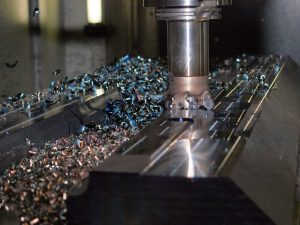Select Page

Cutting speed

Cutting speed is defined as the rate at which the material is removed from the workpiece during machining. In simple terms cutting speed is the metal removal rate. The unit of cutting speed is meters per minute (m/min).In a turning operation during metal removal, for each rotation of the spindle the material along the circumference is removed. This can be denoted as πD.

Cutting speed = πD for one complete rotation. Similarly for two rotations it is going to be 2πD and so on. Therefore for “n” number of rotations Cutting Speed can be denoted as πDN. Where π is a constant, D stands for Diameter of the part / Tool, N stands for number of revolutions in rpm.

As indicated above the unit for cutting speed is meters per minute(m/min). Therefore in case if the  diameter of the part or tool is measured in mm the formula for the cutting speed will be as below

Cutting Speed = πDN / 1000  m/min.

π = 3.14, d = diameter of the part/tool, n= number of revolutions (rpm)

To keep the cutting speed constant while machining various diameters, the spindle rpm is varied.

Spindle speed (n) = C.S x 1000 / π x D  rpm

CS = cutting speed, π = 3.14, d = diameter of the part/tool

Example
Find the cutting speed, when a cylinder of 25 mm diameter is machined at 4500 rpm.

The formula to find the cutting speed is = πDN / 1000  m/min.

3.14 x 25 x 4500
= ———————— m/min
1000

Cutting speed = 353.25 m/min

You can calculate cutting Speed for your requirements below

Find the rpm to turn a cylinder of 20 mm diameter with a cutting speed of 120 m/min.
Diameter of job (d) = 20 mm
Cutting speed (CS) = 120 m/min

The formula to find the rpm (n) = C.S x 1000 / π x D  rpm

120 x 1000
= ——————– rpm
3.14 x 20

Spindle speed = 1910. 82 rpm

You can calculate Spindle Speed for your requirements below

Well i hope you would have got some insights about what Cutting Speed is and how about calculating the cutting speed for and efficient machining process. Kindly let us know your comments in the comment box below. Also if you have any questions feel free to write to us.